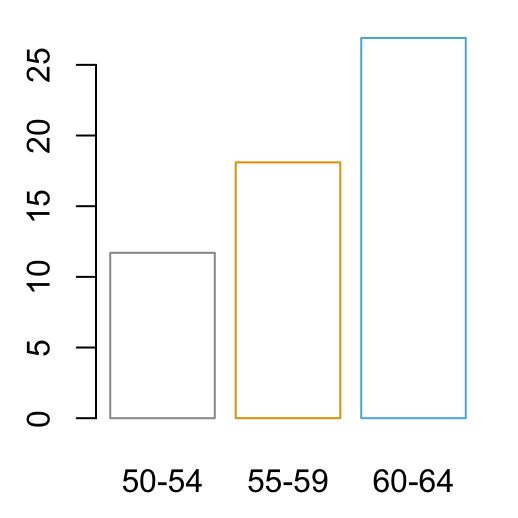R Base Graphs

Previously, we described the essentials of R programming and provided quick start guides for importing data into R.

This chapter contains articles describring how to visualize data using R base graphs.

Creating and saving graphs

• Creating graphs
• Saving graphs
• File formats for exporting plotscreate and save plots

Read more: —> Creating and Saving Graphs in R.

Generic plot types in RRead more: —> Generic plot types in R.

Scatter plots

• R base scatter plot: plot()
• Enhanced scatter plots: car::scatterplot()
• 3D scatter plotsScatter plot matrices

• R base scatter plot matrices: pairs()
• Use the R package psychRead more —> Scatter Plot Matrices.

Box plots

• R base box plots: boxplot()
• Box plot with the number of observations: gplots::boxplot2()Strip Charts: 1-D scatter PlotsRead more —> Strip Charts: 1-D scatter Plots.

Bar plots

• Basic bar plots
• Change group names
• Change color
• Change main title and axis labels
• Stacked bar plots
• Grouped bar plotsLine plots

• R base functions: plot() and lines()
• Basic line plots
• Plots with multiple linesPie charts

• Create basic pie charts: pie()
• Create 3D pie charts: plotix::pie3D()Histogram and density plots

• Create histogram plots: hist()
• Create density plots: density()
##  48.96467 56.38715 60.42221 43.27151 57.14562 57.53028Read more —> Histogram and Density Plots.

QQ-plots: Quantile-Quantile plots

Use qqPlot() [in car package]:Read more —> QQ-plots: Quantile-Quantile plots.

Dot charts

• R base function: dotchart()
• Dot chart of one numeric vector
• Dot chart of a matrixPlot group means and confidence intervalsRead more —> Plot Group Means and Confidence Intervals.

R base graphical parameters

• Add an axis to a plot
• Change axis scale : minimum, maximum and log scale
• Customize tick mark labels
• Change plotting symbols
• Change line types
• Change colors

Read more —> R base graphical Parameters.

Infos

This analysis has been performed using R statistical software (ver. 3.2.4).